## 简介

“Splay” 是 LCT 的基础，但 LCT 中的 Splay 在细节处不太一样，进行了一定的扩展。

## 问题引入

• 修改两点间路径权值
• 查询两点间路径权值和
• 查询子树权值和
• 修改子树权值
• 断开并连接一些边，保证仍然是一棵树

• 维护一个森林，支持删边、加边，保证操作后仍是森林，维护有关信息。
• 一般的操作有两点连通性、两点路径权值和、连接两点、切断某条边、修改信息等。

• 对整棵树按子树大小进行剖分，重新标号。
• 重新标号后，在树上形成了若干以链为单位的连续区间，可以用支持区间操作的数据结构进行修改。

## LCT

### 辅助树(Auxiliary Tree)

#### 性质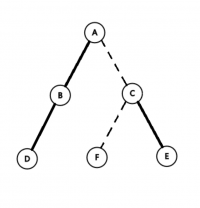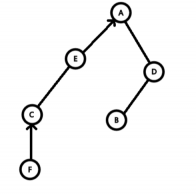### 实现

• to[maxn]，左右儿子。
• fa[maxn]，父亲。
• sum[maxn]，路径权值和，即 Splay 中的子树权值和。
• val[maxn]，点权。
• tag[maxn]，翻转标记。
• lazy[maxn]，权值标记，对应区间加。
• siz[maxn]，辅助树上子树大小
• ……

• pushup(x)，上推操作，维护信息。
• pushdown(x)，下推操作，维护信息。
• get(x)，对应 Splay 中获取父子信息，即获取 $x$ 是父亲的哪个儿子。
• rotate(x)，Splay 中的旋转操作，将 $x$ 向上旋转一层。
• splay(x)，Splay 中的 Splay 操作，将 $x$ 旋转到根。
• access(x)，将原树上根到 $x$ 中的所有点放在一条实链中，使根到 $x$ 成为一条实链，并且在同一棵 Splay 中。本操作是 LCT 的核心
• isroot(x)，判断 $x$ 是否是所在的 Splay 的根。
• update(x)，在 access 操作后，递归地从上到下 pushdown 更新信息。
• makeroot(x)，使 $x$ 成为其所在的树的根。
• link(x,y)，在 $x$ 点与 $y$ 点之间加一条边。
• cut(x,y)，删除 $x$ 点与 $y$ 点之间的边。
• find(x)，找到 $x$ 所在的树的根节点编号。
• fix(x,k)，将 $x$ 的点权修改为 $k$ .
• split(x,y)，提取出 $x$ 点与 $y$ 点之间的路径，方便区间操作。

### 函数详解

#### pushup

 1 2 3 4 5  inline void pushup(int p) { // maintain other variables siz[p] = siz[ls] + siz[rs] + 1; } 

#### pushdown

 1 2 3 4 5 6 7 8  inline void pushdown(int p) { if (tag[p] != std_tag) { // pushdown the tag tag[p] = std_tag; } } 

#### get

 1  inline bool get(int x) { return to[fa[x]] == x; } 

#### isRoot

 1  inline bool isroot(int x) { return to[fa[x]] != x && to[fa[x]] != x; } 

#### rotate

 1 2 3 4 5 6 7 8  void rotate(int x) { int f = fa[x], ff = fa[f], k = get(x); if (!isroot(f)) to[ff][get(f)] = x; //这句一定要写在前面，因为 isroot 的判断机制 to[f][k] = to[x][k ^ 1], fa[to[x][k ^ 1]] = f; to[x][k ^ 1] = f, fa[f] = x, fa[x] = ff; pushup(f), pushup(x); } 

#### splay

 1 2 3 4 5 6  void splay(int x) { update(x); //先下传标记，因为 splay 操作是自下向上的，上方的标记需要先 pushdown 下来 for (int f = fa[x]; !isroot(x); rotate(x), f = fa[x]) if (!isroot(f)) rotate(get(x) == get(f) ? f : x); } 

#### access

 1 2 3 4 5 6  int access(int x) { int p = 0; for (; x; p = x, x = fa[x]) splay(x), rs = p, pushup(x); return p; } 

• 将当前指针节点旋转到根。
• 把儿子换成之前的节点。
• 更新当前点的信息。
• 指针向上。

• 连续两次 access 操作时，第二次操作的返回值是这两个节点的 LCA.
• 表示 $x$ 到根的链所在的 Splay 的根。这个节点一定已经被旋转到了根节点，且父亲一定为空。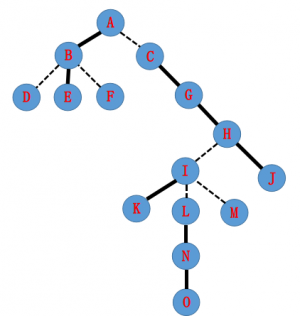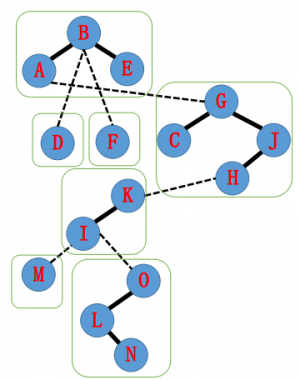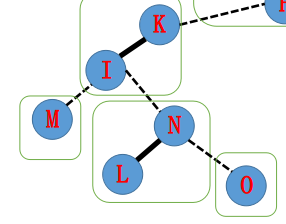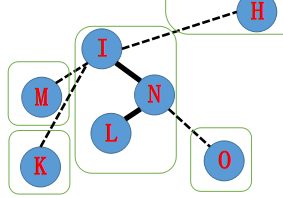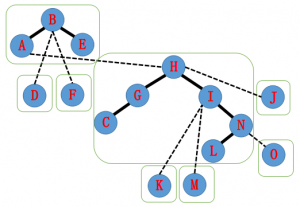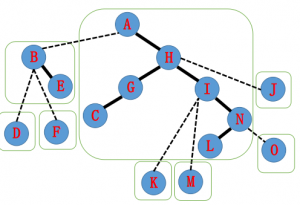• 一棵 Splay 的根到其父亲的边，一定对应原树上的一条虚边。
• 那么一棵 Splay 的根的父亲就是其在原树上的父亲。
• 我们跳到这条实链的顶端节点的父亲上，并将原先的虚边变成实边，依次操作，就能连接出一条实链。
• 为什么连接右儿子？因为根据辅助树的性质，其中序遍历对应原树上的遍历，那么原树上的儿子应该当辅助树上的右儿子。

#### update

 1 2 3 4 5  void update(int x) { if (!isroot(x)) update(fa[x]); pushdown(x); } 

#### makeroot

access 的返回值为 $y$ ，此时 $x$ 到根的路径恰好构成一棵 Splay.

 1  void makeroot(int x) { access(x), splay(x), rev[x] ^= 1; } 

 1  void link(int x, int y) { makeroot(x), splay(x), fa[x] = y; } 

#### split

makeroot(x)，然后 access(y)，就可以得到对应的路径了。 如果需要 $y$ 做根，还可以 splay(y).

#### cut

 1  void cut(int x, int y) { split(x, y), to[y] = fa[x] = 0; } 

• $x$ 与 $y$ 连通。
• $x$ 与 $y$ 路径上没有其他链。
• $x$ 没有右儿子。

 1  void cut(int x, int y) { makeroot(x), access(y), splay(y); if (!to[x] && to[y] == x) fa[x] = to[y] = 0; } 

#### find

access(x) 后，再 splay(x)，这样根就是该 Splay 中的最小值，根据"二叉搜索树"性质，一路走左儿子即可。

 1 2 3 4 5 6  int find(int x) { access(x), splay(x); while (ls) pushdown(x), x = ls; return splay(x), x; } 

#### fix

 1  access(x), splay(x), val[x] = y, pushup(x); 

### 注意

• 一定记得 pushuppushdown，非常重要。
• LCT 中的 rotate 需要先判断 isroot，所以连接父亲的父亲的关系的语句在最前面。
• LCT 中的 splay 就是旋转到根，不需要旋转到儿子。
• 建树时，可以默认所有边为虚边，直接只指定父亲关系。

#### pushdown 翻转

  1 2 3 4 5 6 7 8 9 10 11 12  inline void pushdown(int x) { if (!rev[x]) return; std::swap(ls, rs), rev[ls] ^= 1, rev[rs] ^= 1, rev[x] = 0; } int nex(int x) { pushdown(x); if (rs) x = rs; again: pushdown(x); if (ls) { x = ls; goto again; } return splay(x), x; } 

### 维护树链信息

LCT 通过 “split” 操作，可以将树上 $x$ 点到 $y$ 点的路径提取到一棵 Splay 中，树链信息的修改和统计转化为平衡树上的操作，这让 LCT 在维护树链信息上具有优势。

### 维护边权

LCT 不能直接处理边权，因此需要利用虚点来维护边权。

LCT 不擅长维护子树信息。